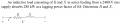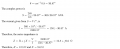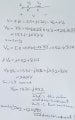# circuit analysis

#### skyline977

Joined Jan 14, 2021
29
Hi all

While solving the below circuit:The solution is as shown below:I noticed the following:My question is how for a pure resistance both the voltage across it and current through it have imaginary parts?
What do these imaginary parts represent?
Similarly for a pure reactive load (inductive) what does the real part of the voltage Vx mean?

#### neonstrobe

Joined May 15, 2009
180
The point is that in a resistor, there is only a real value for voltage and current. The "imaginary" part is that the current in the pair is out of phase with the voltage. That current can be resolved (Pythagoras?) into two parts: a real bit (which is what you see across the resistor ) and an only fully imaginary part (which you see across the inductor). The net effect of voltage and current being on different phases arises across the pair.
The reason for using complex numbers is that a phase shift can be mapped to the imaginary axis as it is at 90 degrees to the real axis.
Voltages and currents you calculate across a pure reactive impedance are what you would measure, but unless you measure the phase specifically you won't see that on a meter (unless it can measure phase with respect to some other input).
Adding in edit - so the voltage across a resistor should only have real values an and the current in a pure reactance should only be the 90-degrees imaginary current to the voltage across it.
Just to add another clue - the voltage you calculated indicates a phase shift relative to the applied voltage. You need the resultant (Pythagoras) to calculate the resultant voltage across the resistor. Likewise with the current.
Final clue - think about what the effective current is, given the real and imaginary parts. Plot on an Argand diagram (complex graph). You are nearly there, and just need the final push.

Last edited:
•skyline977